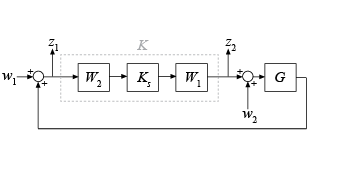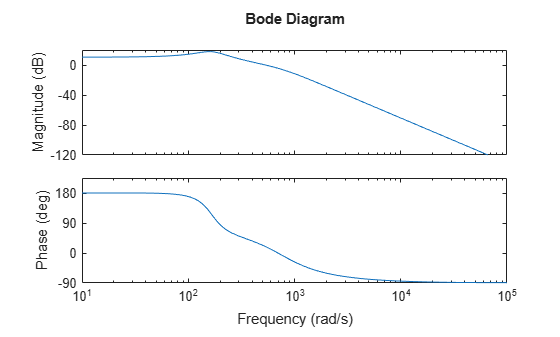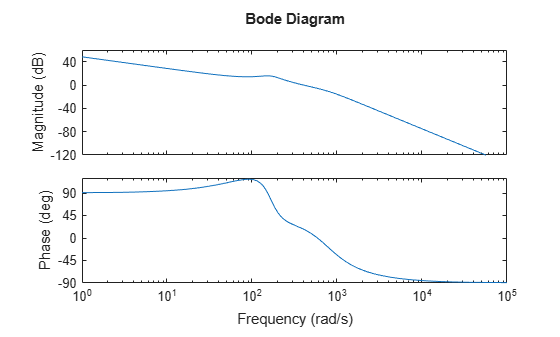ncfsyn

Loop shaping design using Glover-McFarlane method

Description

ncfsyn implements a method for designing controllers that uses a combination of loop shaping and robust stabilization as proposed in -. The function computes the Glover-McFarlane H normalized coprime factor loop-shaping controller K for a plant G with pre-compensator and post-compensator weights W1 and W2. The function assumes the positive feedback configuration of the following illustration.To specify negative feedback, replace G by –G. The controller Ks stabilizes a family of systems given by a ball of uncertainty in the normalized coprime factors of the shaped plant Gs = W2GW1. The final controller K returned by ncfsyn is obtained as K = W1KsW2.

[K,CL,gamma,info] = ncfsyn(G) computes the Glover-McFarlane H normalized coprime factor loop-shaping controller K for the plant G, with W1 = W2 = I. CL is the closed-loop system from the disturbances w1 and w2 to the outputs z1 and z2. The function also returns the H performance gamma, and a structure containing additional information about the result.

example

[K,CL,gamma,info] = ncfsyn(G,W1) computes the controller using the pre-compensator weight you specify in W1, with W2 = I.

[K,CL,gamma,info] = ncfsyn(G,W1,W2) computes the controller using the specified pre-compensator weight W1 and post-compensator weight W2.

example

[K,CL,gamma,info] = ncfsyn(G,W1,W2,tol) specifies how tightly the performance gamma of the synthesized controller approximates the optimal achievable performance gopt. Increasing tol can help when the controller K has undesirable fast dynamics.

Examples

collapse all

Use ncfsyn to design a controller for the following plant.

G = tf([1 5],[1 2 10]);

Use weighting functions that yield a shaped plant W1*G*W2 with high gain for disturbance attenuation below 0.1 rad/s, and low gain for good robust stability above about 5 rad/s. For this G, a pre-compensator weight alone is sufficient.

W1 = tf(1,[1 0]);
bodemag(W1*G)
gridCompute the controller.

[K,CL,gamma,info] = ncfsyn(G,W1);

The optimal cost gamma is related to the normalized coprime stability margin of the system by 1/gamma = ncfmargin(Gs,-Ks). (The minus sign is needed because ncfmargin assumes a negative-feedback loop, while ncfsyn computes a positive-feedback controller.)

b = ncfmargin(info.Gs,-info.Ks);
[gamma 1/b]
ans = 1×2

1.4521    1.4521

Compare the achieved and target loop shapes.

sigma(G*K,G*W1)
legend('achieved','target')In the controller returned by ncfsyn, some controller poles can become infinitely fast as the actual performance gamma approaches the optimal achievable performance gopt. To prevent this, check the controller poles and increase the tol argument if some poles are undesirably fast. tol sets how closely gamma of the synthesized controller approximates gopt.

bode(G)Use a weighting function that yields a shaped plant W1*G with high gain for disturbance attenuation below about 10 rad/s, and low gain for good robust stability above about 1000 rad/s.

W1 = zpk(-130,0,0.6);
bode(W1*G)Design a controller using the default tolerance, tol = 1e-3. Examine the poles of the resulting controller.

[K1,~,gamma1,info1] = ncfsyn(G,W1);
damp(K1)

Pole              Damping       Frequency      Time Constant

0.00e+00                -1.00e+00       0.00e+00              Inf
-1.48e+02 + 1.60e+01i     9.94e-01       1.49e+02         6.76e-03
-1.48e+02 - 1.60e+01i     9.94e-01       1.49e+02         6.76e-03
-7.90e+02 + 8.01e+02i     7.02e-01       1.13e+03         1.27e-03
-7.90e+02 - 8.01e+02i     7.02e-01       1.13e+03         1.27e-03
-1.50e+05                 1.00e+00       1.50e+05         6.66e-06

This controller has a pole at around 150000 rad/s, much faster than any other dynamics in the controller. To reduce the frequency of this pole, try increasing the tolerance to 0.1.

tol = 0.1;
[K2,~,gamma2,info2] = ncfsyn(G,W1,[],tol);
damp(K2)

Pole              Damping       Frequency      Time Constant

0.00e+00                -1.00e+00       0.00e+00              Inf
-1.50e+02 + 6.85e+00i     9.99e-01       1.50e+02         6.68e-03
-1.50e+02 - 6.85e+00i     9.99e-01       1.50e+02         6.68e-03
-6.58e+02 + 9.45e+02i     5.71e-01       1.15e+03         1.52e-03
-6.58e+02 - 9.45e+02i     5.71e-01       1.15e+03         1.52e-03
-1.77e+03                 1.00e+00       1.77e+03         5.65e-04

This time the highest-frequency pole is closer to the others. Compare the resulting performance values to confirm that the two controllers deliver similar performance.

gamma1, gamma2
gamma1 = 2.1157
gamma2 = 2.3250

Input Arguments

collapse all

Plant, specified as a dynamic system model such as a state-space (ss) model. If G is a generalized state-space model with uncertain or tunable control design blocks, then ncfsyn uses the nominal or current value of those elements. G must have the same number of inputs and outputs.

Pre-compensator weight, specified as:

• Identity matrix eye(N), where N is the number of inputs or outputs in G.

• SISO minimum-phase LTI model. In this case, ncfsyn uses the same weight for every loop channel.

• MIMO minimum-phase LTI model of the same I/O dimensions as G.

Select pre-compensator and post-compensator weights W1 and W2 such that the gain of the shaped plant Gs = W2GW1 is sufficiently high at frequencies where good disturbance attenuation is required, and sufficiently low at frequencies where good robust stability is required.

Post-compensator weight, specified as the identity matrix eye(N) or a SISO or MIMO LTI model. The considerations for specifying W2 are the same as those for W1. To omit the post-compensator weight, set W2 = [].

Near-optimality tolerance, specified as a positive scalar value. ncfsyn returns a controller K with performance gamma that satisfies abs(gamma-gopt) < tol*gopt, where gopt is the optimal performance (returned in info). Use tol to adjust the acceptable gap between gamma and gopt. A tol value that is too small can cause numerical difficulties and introduce fast poles in K. Increasing tol usually eliminates both issues. For an example, see Reduce Undesirable Fast Dynamics in Controller.

Output Arguments

collapse all

H-optimal loop-shaping controller, returned as a state-space (ss) model with the same I/O dimensions as G. The optimal controller K = W1KsW2. See Algorithms.

Optimal closed-loop system from the disturbances w1 and w2 to the outputs z1 and z2, returned as a state-space model. The closed-loop system is given by:

$\left[\begin{array}{c}I\\ K\end{array}\right]{\left(I-GK\right)}^{-1}\left[I,\text{\hspace{0.17em}}G\right].$

H performance achieved with the controller K, returned as a positive scalar value greater than 1. The H performance is hinfnorm(CL). The optimal controller Ks is such that the singular-value plot of the shaped loop Ls = W2GW1Ks optimally matches the target loop shape Gs to within a factor of gamma. However, for numerical reasons, ncfsyn generally returns a controller with slightly larger H performance than optimal. For the optimal achievable performance, see the info output argument.

gamma is related to the normalized coprime stability margin of the system by gamma = 1/ncfmargin(Gs,-Ks). Thus, gamma gives a good indication of robustness of stability to a wide class of unstructured plant variations, with values in the range 1 < gamma < 3 corresponding to satisfactory stability margins for most typical control system designs.

Additional information about the controller synthesis, returned as a structure containing the following fields.

• gopt — Optimal performance achievable by H synthesis for the shaped plant. For numerical reasons, ncfsyn generally returns a controller with slightly larger H performance, which is returned in gamma. To adjust how tightly ncfsyn aims to make gamma match gopt, use the tol input argument. See Reduce Undesirable Fast Dynamics in Controller.

• emaxnugap robustness metric, emax = 1/gopt (see gapmetric)

• Gs — Shaped plant Gs = W2GW1

• Ks — Optimal controller for shaped plant Gs. The final controller is K = W1KsW2. See Algorithms for details.

Tips

• While ncfmargin assumes a negative-feedback loop, the ncfsyn command designs a controller for a positive-feedback loop. Therefore, to compute the margin using a controller designed with ncfsyn, use [marg,freq] = ncfmargin(G,K,+1).

Algorithms

The returned controller K = W1KsW2, where Ks is an optimal H controller that minimizes the H cost

$\gamma \left({K}_{s}\right)={‖\left[\begin{array}{c}I\\ {K}_{s}\end{array}\right]{\left(I-{G}_{s}{K}_{s}\right)}^{-1}\left[I,{G}_{s}\right]‖}_{\infty }={‖\left[\begin{array}{c}I\\ {G}_{s}\end{array}\right]{\left(I-{K}_{s}{G}_{s}\right)}^{-1}\left[I,{K}_{s}\right]‖}_{\infty }.$

The optimal performance is the minimal cost

$\gamma :=\underset{{K}_{s}}{\mathrm{min}}\gamma \left({K}_{s}\right).$

Suppose that Gs=NM–1, where N()*N() + M()*M() = I, is a normalized coprime factorization (NCF) of the weighted plant model Gs. Then, theory ensures that the control system remains robustly stable for any perturbation ${\stackrel{˜}{G}}_{s}$ to Gs of the form

${\stackrel{˜}{G}}_{s}=\left(N+{\Delta }_{1}\right){\left(M+{\Delta }_{2}\right)}^{-1}$

where Δ1, Δ2 are a stable pair satisfying

${‖\left[\begin{array}{c}{\Delta }_{1}\\ {\Delta }_{2}\end{array}\right]‖}_{\infty }

The closed-loop H-norm objective has the standard signal gain interpretation. Finally it can be shown that the controller, Ks, does not substantially affect the loop shape in frequencies where the gain of W2GW1 is either high or low, and will guarantee satisfactory stability margins in the frequency region of gain cross-over. In the regulator set-up, the final controller to be implemented is K=W1KsW2.

See McFarlane and Glover  for details.

Compatibility Considerations

expand all

Not recommended starting in R2020b

 McFarlane, Duncan C., and Keith Glover, eds. Robust Controller Design using Normalized Coprime Factor Plant Descriptions. Vol. 138. Lecture Notes in Control and Information Sciences. Berlin/Heidelberg: Springer-Verlag, 1990. https://doi.org/10.1007/BFb0043199.

 McFarlane, D., and K. Glover, “A Loop Shaping Design Procedure using H Synthesis,” IEEE Transactions on Automatic Control, no. 6 (June 1992): pp. 759–69. https://doi.org/10.1109/9.256330.

 Vinnicombe, Glenn. “Measuring Robustness of Feedback Systems.” PhD Dissertation, University of Cambridge, 1992.

 Zhou, Kemin, and John Comstock Doyle. Essentials of Robust Control. Upper Saddle River, NJ: Prentice-Hall, 1998.

Robust Control Toolbox DocumentationGet trial now